\$B:#>,\$N0lGU(B

\$BKhF|!":#>,\$N0lGU\$r>e\$2\$F\$\$\$^\$9!#(B
\$B:#>,0{\$`\$b\$N\$K:\$\$C\$?\$i!";29M\$K\$7\$F\$_\$F\$/\$@\$5\$\$!#(B

2019\$BG/(B11\$B7n(B

 1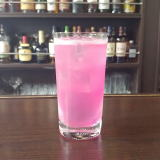(IK^8DX0[0D^(B 2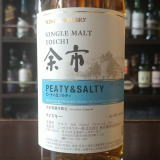\$BM>;T(IK_0C(0(B&(I?YC(0(B 3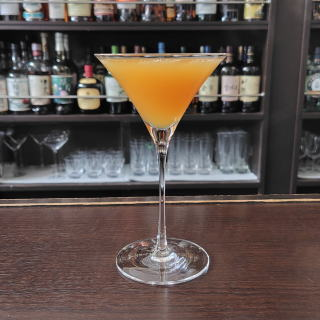(I6Y3^'D^=%68CY(B 4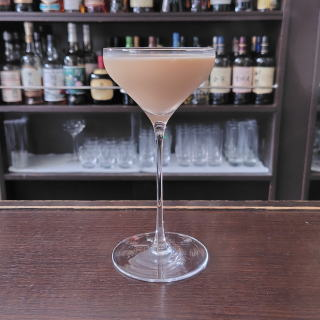(IL_X]>=%R1X0(B 5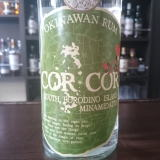(I:Y:Y(B 6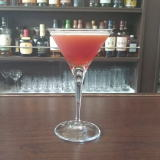(I@26^0=%PY8(B 7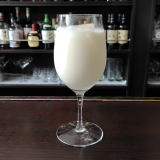(IIA*[%PY8(B 8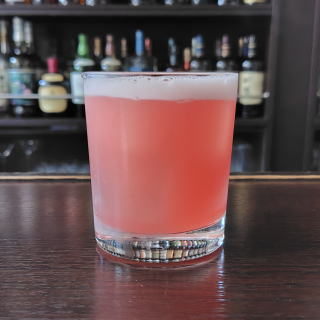(IL_WDF/8%WL^(B 9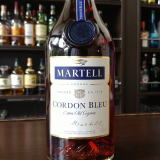(IO0CY\$B!!(I:YD^]L^Y0(B 10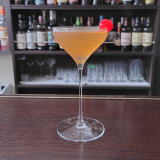(I32=70%68CY(B 11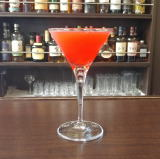(I:]J_D^0Z(B 12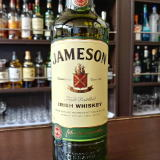(I<^*2Q?](B 13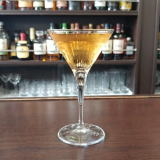(IN/L_=:/A(B 14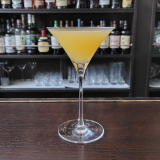(I:^0YC^]%C^2=^(B 15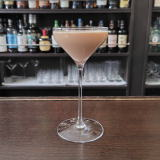(I1L@0\$B#8(B 16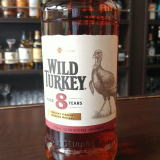(I\2YD^@070(B8\$BG/(B 17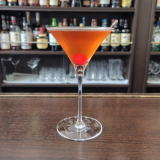(IJ_0L*8D%OC(0F(B 18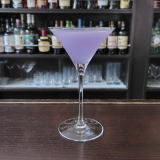(IL'2EY%1L_[0A(B 19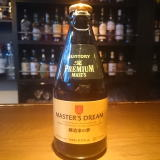(IO=@0=^%D^X0Q(B 20(IK_40Y%:X]=^(B 21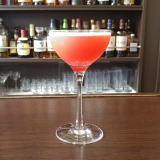(IL']L'0Z(B 22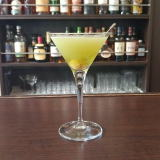(I4L^XN^C^(=^12X/<-(B 23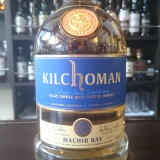(I7YN0O](B (IO710M^2(B 24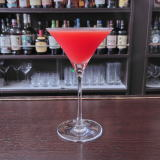(I@^F4Y%68CY(B 25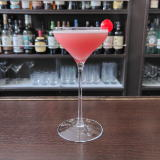(IA16^0Y(B 26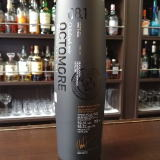(I58DS1(B 27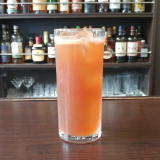(IC^;^0D%K0W0(B 28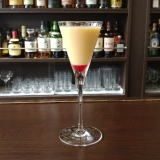(I<^,O26%<^.0(B 29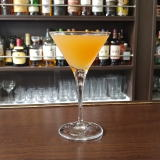\$B%3%K%c%C%/!&%+%/%F%k(B 30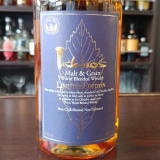(I2A[0=^SYD(B&(I8^Z0](B